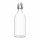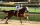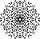# Fraction calculator

The calculator performs basic and advanced operations with fractions, expressions with fractions combined with integers, decimals, and mixed numbers. It also shows detailed step-by-step information about the fraction calculation procedure. Solve problems with two, three, or more fractions and numbers in one expression.

## Result:

### (7/8) : (1/20) = 35/2 = 17 1/2 = 17.5

Spelled result in words is thirty-five halfs (or seventeen and one half).

### How do you solve fractions step by step?

1. Divide: 7/8 : 1/20 = 7/8 · 20/1 = 7 · 20/8 · 1 = 140/8 = 4 · 35 /4 · 2 = 35/2
Dividing two fractions is the same as multiplying the first fraction by the reciprocal value of the second fraction. The first sub-step is to find the reciprocal (reverse the numerator and denominator, reciprocal of 1/20 is 20/1) of the second fraction. Next, multiply the two numerators. Then, multiply the two denominators. In the following intermediate step, cancel by a common factor of 4 gives 35/2.
In other words - seven eighths divided by one twentieth = thirty-five halfs.

#### Rules for expressions with fractions:

Fractions - simply use a forward slash between the numerator and denominator, i.e., for five-hundredths, enter 5/100. If you are using mixed numbers, be sure to leave a single space between the whole and fraction part.
The slash separates the numerator (number above a fraction line) and denominator (number below).

Mixed numerals (mixed fractions or mixed numbers) write as integer separated by one space and fraction i.e., 1 2/3 (having the same sign). An example of a negative mixed fraction: -5 1/2.
Because slash is both signs for fraction line and division, we recommended use colon (:) as the operator of division fractions i.e., 1/2 : 3.

Decimals (decimal numbers) enter with a decimal point . and they are automatically converted to fractions - i.e. 1.45.

The colon : and slash / is the symbol of division. Can be used to divide mixed numbers 1 2/3 : 4 3/8 or can be used for write complex fractions i.e. 1/2 : 1/3.
An asterisk * or × is the symbol for multiplication.
Plus + is addition, minus sign - is subtraction and ()[] is mathematical parentheses.
The exponentiation/power symbol is ^ - for example: (7/8-4/5)^2 = (7/8-4/5)2

#### Examples:

subtracting fractions: 2/3 - 1/2
multiplying fractions: 7/8 * 3/9
dividing Fractions: 1/2 : 3/4
exponentiation of fraction: 3/5^3
fractional exponents: 16 ^ 1/2
adding fractions and mixed numbers: 8/5 + 6 2/7
dividing integer and fraction: 5 ÷ 1/2
complex fractions: 5/8 : 2 2/3
decimal to fraction: 0.625
Fraction to Decimal: 1/4
Fraction to Percent: 1/8 %
comparing fractions: 1/4 2/3
multiplying a fraction by a whole number: 6 * 3/4
square root of a fraction: sqrt(1/16)
reducing or simplifying the fraction (simplification) - dividing the numerator and denominator of a fraction by the same non-zero number - equivalent fraction: 4/22
expression with brackets: 1/3 * (1/2 - 3 3/8)
compound fraction: 3/4 of 5/7
fractions multiple: 2/3 of 3/5
divide to find the quotient: 3/5 ÷ 2/3

The calculator follows well-known rules for order of operations. The most common mnemonics for remembering this order of operations are:
PEMDAS - Parentheses, Exponents, Multiplication, Division, Addition, Subtraction.
BEDMAS - Brackets, Exponents, Division, Multiplication, Addition, Subtraction
BODMAS - Brackets, Of or Order, Division, Multiplication, Addition, Subtraction.
GEMDAS - Grouping Symbols - brackets (){}, Exponents, Multiplication, Division, Addition, Subtraction.
Be careful, always do multiplication and division before addition and subtraction. Some operators (+ and -) and (* and /) has the same priority and then must evaluate from left to right.

## Fractions in word problems:

• Ten fractionsWrite ten fractions between 1/3 and 2/3
• DivideDivide the number 72 in the ratio 7: 2 and calculate the ratio of the numbers found in this order and write down as decimal.
• Collection of stampsJano, Rado, and Fero have created a collection of stamps in a ratio of 5: 6: 9. Two of them had 429 stamps together. How many stamps did their shared collection have?
• Youth trackYouth track from Hronská Dúbrava to Banská Štiavnica which announced cancellation attracted considerable media attention and public opposition, has cost 6.3 euro per capita and revenue 13 cents per capita. Calculate the size of subsidies to a trip group o
• Pie division5/8 of a pie divide 6 pieces. Each friend got 1/6.  What fraction  of the whole pie does each person receive?
• How many 16How many three-tenths are there in two and one-fourths?
• A PVC pipeA PVC pipe 12 feet long is to be cut into 1 1/2 foot pieces.  How many pieces can a plumber get out of this length pipe?
• Kate sharesKate shares a 64-ounce bottle of apple cider with five friends. Each person's serving will be the same number of ounces. Between what two whole number of ounces will each person's serving to be? Explain using division.
• Golf ballsOf the 28 golf balls, 1/7 are yellow. How many golf balls are yellow? Use the model to help you. Enter your answer in the box.
• A farmer's heritageA farmer died leaving his 17 horses to his 3 sons. When his sons opened up the Will it read: My eldest son should get 1/2 (half) of total horses; My middle son should be given 1/3rd (one-third) of the total horses; My youngest son should be given 1/9th (o
• What fractionWhat fraction of number 1 to 30 is prime?
• Each ofEach of Suzan's notebooks is 4/5  inches wide. If she has 25 inches of space remaining on her bookshelf, how many notebooks will fit?
• Metal rodYou have a metal rod that’s 51/64 inches long. The rod needs to be trimmed. You cut 1/64 inches from one end and 1/32 inches from the other end. Next, you cut the rod into 6 equal pieces. What will be the final length of each piece?